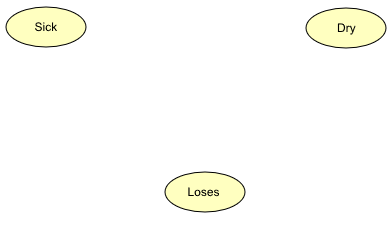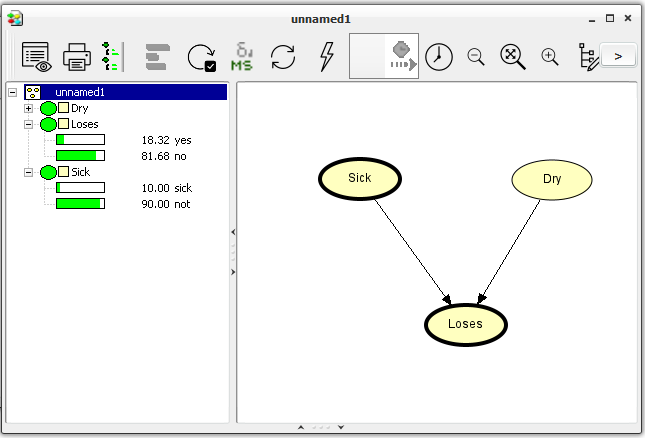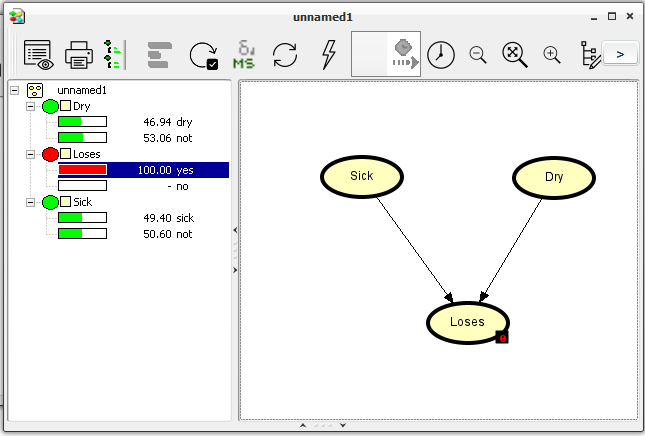# How to Build a Bayesian Network¶

This tutorial shows you how to implement a small Bayesian network in the Hugin Graphical User Interface. The network we are about to implement is the one modeled in the Apple Tree example in the Bayesian Networks Tutorial.

The qualitative representation of our network is shown in Figure 1.

If you want to understand the design of this network, you should read about it in the Bayesian Networks Tutorial.

## Constructing a New Network¶

When you choose to start up the HUGIN Graphical User Interface, the Main HUGIN Window (or simply the Main Window) opens. This window contains a menu bar (called the Main Window Menu Bar), a tool bar (called the Main Window Tool Bar), and a document pane (called the Main Window Document Pane or simply the Document Pane). In the Document Pane, a new empty network called “unnamed1” is automatically opened in a network window (see Figure 2). It starts up in Edit Mode which allows you to start constructing the network immediately (the other main mode is Run Mode which allow you to use the network).Figure 2: The network window containing a Tool Bar, a Tables Pane, and a Network Pane.¶

The first thing we will do is add the Sick node. This can be done as follows:

• Select the Discrete Chance Tool in the Tool Bar of the “unnamed1” network window (see Figure 3).

• Click somewhere in the Network Pane (see Figure 2).

When we have clicked in the Network Pane, a node labeled “C1” appears. We want to change this label to “Sick”:

• Select the node with the mouse cursor.

• Enter “Node Properties” by pressing the node properties tool (see Figure 3).

• Change both the “Name” and the “Label” fields to “Sick”.

• Press the “OK” button.

The “Name” is the internal name of the node while “Label” is the label of the node. If no label is specified (as was the case before we changed the label) the label used is the internal name. The internal name can consist of only the letters ‘a’-‘z’ and ‘A’-‘Z’, the digits ‘0’-‘9’, and the underscore character ‘_’ while the label can be almost anything. Please note that the first character of the name must be a letter.

•: The Discrete Chance tool

•: The Node Properties tool

•: The Link tool

The Dry and Loses nodes are added the same way. We can add more nodes without having to press the Discrete Chance Tool all the time by holding down the SHIFT key while clicking in the Network Pane. When we have chosen a node in the Network Pane, we can access the node properties tool by holding down the right mouse button.Figure 4: The Network pane contains the three nodes Sick, Dry, and Loses that have been added to the network.¶

### The States¶

In the introduction to BNs the states of the nodes were specified as follows: Sick has two states: “sick” and “not”, Dry has two states: “dry” and “not”, and Loses has two states “yes” and “no”.

First, we open the Tables Pane by clicking the tables-pane button.Figure 5: The CPT is opened by pressing the left mouse button over a node, while holding down the “ctrl” button.¶

Next, we specify the states of Sick:

• Hold down the “ctrl” button while clicking the left mouse button over the node “Sick” to display its CPT in the Tables Pane, as shown in Figure 5.

• Click the field containing the text “State 1” in the CPT in the Tables Pane.

• Type the text “sick” in the field to give the state this name.

• Click the field containing the text “State 2” in the CPT.

• Type the text “not” in the field.

Now, do the same with Dry.

We can do exactly the same with Loses. Beware that the CPT of Loses is a little bigger than those of Sick and Dry. This is just because Loses has parent nodes (Sick and Dry don’t).

• Name the two states of Loses “yes” and “no”.

### Entering CPT Values¶

The next step is to enter the CPT values correctly (as default, the Hugin Graphical User Interface has given all nodes a uniform distribution). The values were specified in the introduction to BNs and they are shown in Tables 1, 2, and 3.

First, we select all three nodes (shortcut: Ctrl+A) to get the CPTs displayed in the Tables Pane. Next, we enter the values into the Sick node:

• Click the field representing Sick=”sick”.

• Enter the value 0.1 (from Table 1).

• Click the field representing Sick=”not”.

• Enter the value 0.9 (from Table 1).

Enter the values for Dry and Loses the same way. When you have entered the CPT for Loses, the network window should look like Figure 6.Figure 6: The network window with node Loses selected. The CPT of Loses appears in the Tables Pane.¶

This completes the construction of the network. At this point it would be a good idea to save the network. Here is how to do it:

• Select “Save” (or “Save As”) from the “File” menu.

• Enter a name (e.g. “apple”).

• Press “Save”.

## Compiling the Network¶

Now, let’s compile the network and see how it works:

• Press the Run Mode tool button in the Tool Bar (see Figure 7).

The compiler checks for the following errors:

• Cycles. There must be no directed cycles in a network.

• For each parent configuration of a node the probabilities of the different states must have the sum of 1. In other words, each column of the table must sum to 1. If there is a column that does not sum to 1, the compiler will normalize the values. This fact can be utilized when filling in the probabilities. Say, for example, that the probability of a tree being sick is based on the observation of 13527 trees over one season, where 1678 got sick and the rest didn’t. Instead of first computing the fractions, we just put 1678 in the sick state of the Sick node, and 11849 in the no state. Then the compiler will compute the proper values.

If you have done exactly as this tutorial told you, there should not be any errors in the compilation process. The compilation should be finished very fast with a small network like ours. After the compilation, the Run Mode is entered (we have so far only been working in Edit Mode).

## Running the Network¶

Running in Run Mode, the network window is split into two by a vertical bar (see Figure 8). To the left is the Node List Pane and to the right is the Network Pane.Figure 8: The network window in Run Mode. To the left is the Node List Pane (having Loses and Sick expanded) and to the right is the Network Pane.¶

We can view the probabilities of a node being in a certain state by expanding the node in the Node List Pane. We expand (collapse) a node by clicking its expand (collapse) icon in the Node List Pane, by double-clicking its node symbol in the Node List Pane, or by selecting (deselecting) it in the Network Pane. We can also expand (collapse) all nodes at once by pressing the expand (collapse) node list tool in the Tool Bar just to the right of the node properties tool.

### Is the Tree Sick?¶

Now, imagine that we want to use our network to find the probability of an apple tree being sick given the information that the tree is losing its leaves. This is done as follows:

• Expand all nodes (by pressing the expand node list tool).

• Enter the fact that the tree is losing its leaves by double clicking the state “yes” of the Loses node.

• Propagate this piece of evidence by pressing the Sum Propagation Tool in the Tool Bar (see Figure 9).

• Read the probability of Sick being in state “sick”

This should give the output shown in Figure 10.Figure 10: Our network after the evidence that the tree is losing its leaves has been entered and propagated.¶

The probability of the tree being sick is now 0.49.

If you do not read the value specified above, you have probably mistyped something when filling in the CPTs. Then please check the CPTs of all the nodes.

## The Monitor Windows¶

In the last section, we used the Node List Pane to enter evidence and retrieve beliefs. We can also do this by using the monitor windows. The monitor windows show the same information as the Node List Pane but we have the opportunity to place the monitor windows near the corresponding nodes of the network in the Network Pane. We can open a monitor window for each node in the Network Pane, but the best way to use them is probably only to open a monitor window for the nodes in the network which have special interest. Otherwise, they might take up too much space.

Now, we shall open monitor windows for Sick and Loses and repeat the computations from before. First, initialize the network:

• Press the Initialize Tool button (to the left of the Sum Propagation Tool).

Then, we are ready to open the monitor windows of Sick and loses.

• Select Sick and Loses (hold down the SHIFT key to select more nodes at the same time).

• Choose “Show Monitor Windows” from the “View” menu.Figure 11: Monitor windows of Sick and loses shown in the Network Pane.¶

The rest of this tutorial introduces some very useful aspects of the HUGIN Graphical User Interface, but it can be skipped.

### The Most Likely Combination¶

From the propagation in the previous section we could see that the probability of the apple tree suffering from drought is 0.47. In both the case of Sick and Dry it is more likely that the state is “not”. This could make one believe that the most likely combination of states is when both Sick and Dry are in state “not”. However, this is a wrong conclusion. If we want to find the most likely combination of states in all nodes, we should use max propagation (in stead of sum propagation). The Max Propagation Tool is found in the Tool Bar just to the right of the Sum Propagation Tool.

Now, try to press the Max Propagation Tool. In each node, a state having the value 100.00 belongs to a most likely combination of states. In this case, this gives one unique combination being the most likely: Sick is “sick” and Dry is “not”

We see that even if Sick=”sick” is less likely than Sick=”not”, Sick=”sick” is contained in the most likely combination of the states of the nodes while Sick=”not” is not. This shows that we need to be careful in making conclusions from the result of a propagation.

Now, one might want to know the probability of this most likely combination of states (or of any other combination of states) under the assumption that the entered evidence holds.

### Computing the Probability of a Combination of States¶

Here, we shall describe a technique to compute the probability of the most likely combination of states given the evidence that the apple tree is losing its leaves. This probability is written:

$P(Sick="yes", Dry="not" | Loses="yes")$

Each time we perform sum propagation in a network, the probability of the entered evidence is shown in the lower left corner of the HUGIN Graphical User Interface window (the P(All) value). If we have chosen the “yes” state of the Loses node and performed sum propagation, we can read the probability of Loses=”yes” (written P(Loses=”yes”)). This value should be 0.1832.

The technique uses the following rule from probability theory (known as the fundamental rule):

$P(A, B) = P(A | B) P(B)$

The only kind of probability we can get from HUGIN is the probability of a series of pieces of evidence which can be written in the form:

$P(A1, A2,..., An)$

We use the fundamental rule to rewrite our requested probability to some expression composed by such components:

\begin{align}\begin{aligned}P(Sick="yes", Dry="not" | Loses="yes")\\= P(Sick="sick", Dry="not", Loses="yes") / P(Loses="yes")\end{aligned}\end{align}

In the fundamental rule, we have divided both sides with P(B). Then we have substituted A with Sick=”yes”, Dry=”not” and B with Loses=”yes”.

We already know P(Loses=”yes”) so we only need to compute P(Sick=”sick”, Dry=”not”, Loses=”yes”). This is done as follows:

• Enter Sick=”sick”, Dry=”not”, and Loses=”yes” in the network.

• Press the Sum Propagation Tool.

• Read P(Sick=”sick”, Dry=”not”, Loses=”yes”) as the P(All) value in the lower left corner.

This value should be 0.081. Now, we are ready to compute the requested probability:

\begin{align}\begin{aligned}P(Sick="yes", Dry="not" | Loses="yes")\\= 0.081 / 0.1832\\= 0.442\end{aligned}\end{align}

So, the probability of the most likely combination of states of Sick and Dry, given that Loses=”yes”, is 0.442.